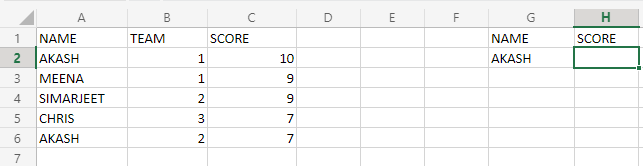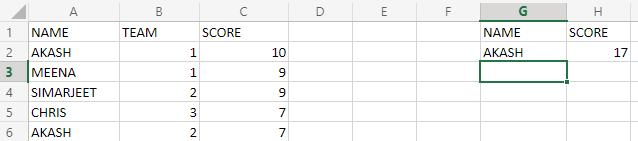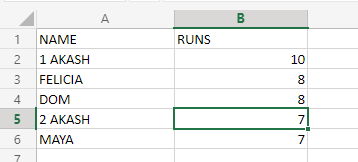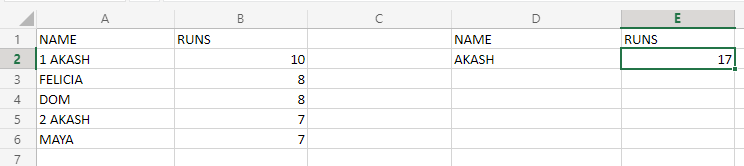# Using the SUMIF Function to Add Cells if They Contain Certain Text

• Last Updated : 06 Sep, 2021

In Excel, sometimes we want to sum up the values based on some specific text values. Such as the cells which have text written as “ME” will be added, so we will see how to achieve this requirement. We can also use VLOOKUP with case sensitivity to achieve the above requirement, but EXCEL provides us a function dedicated to this. So we will use a function called “SUMIF” to implement the requirement.

First, let’s look into the SUMIF function.

Attention reader! Don’t stop learning now. If you are an Excel beginner (or an intermediate) and want to learn Excel, Geeksforgeeks brings the perfect course for you to start, Diving Into Excel

### The SUMIF Function:

SUMIF function can add specific cell(s) values, based on the criteria, or the keyword it will use to filter out the values and add only those cells values, which are having this keyword associated with them.

Syntax:

`SUMIF(range,"crieteria or keyword",cell values to be added)`

Here,

• The range specifies the list of cells on which the criteria will be matched,i.e the cells matching the above keyword, only their value will be considered for the sum.
• The crieteria or keyword highlights the matching keyword, that will be used by the SUMIF function.
• The cell values will be that column in which the values will be picked up for sum. Here the cells which were selected in the range column, only their value will be considered for summing in the result.

Example:

Now take a look at the below image:Here, we consider a dataset that will store individual scores of members of 3 teams. But our requirement is that we want to calculate the score of AKASH because there are 2 AKASH in different teams. So, the arguments of SUMIF function to calculate the score will be–

• The range will be(A2:A6). The criteria will be matched from this column only.
• The criteria will be“AKASH”.
• The sum range will be C2:C6). The values will be picked from this range for summing.

So the formula will be as shown below:

Formula:

`=SUMIF(A2:A6,"AKASH",C2:C6)`

This formula will be applied to the H2 column. So the output will be as shown below:### Using the SUMIF Function on Partial Text:

Now, let’s suppose we don’t want to give criteria in form of a word, or a letter, but rather an excel cell value. So in this case, the formula will get modified, and it will look like below:

Formula:

`=SUMIF(range,"*"&cell_name&"*",sum_range)`

Considering the above dataset only, we will see how the formula will change.

`=SUMIF(A2:A6,"*"&G2&"*",C2:C6)`

Here, we used G2, which is having the value AKASH, which will be used as criteria.

Here the output will be the same as the above case.

Output:### Using SUMIF on TEXT and Numbers:Now, let’s suppose we have this dataset. We get a dataset in which the redundant values, which in this case is AKASH, are distinguished by the prefix numbering. We want to calculate how many runs all AKASH’s scored in the team. So, the SUMIF can help us achieve this, but we will modify the formula just a little bit, to make it compatible with cells that are having numbers and text. The formula will be as follows:

Formula:

`=SUMIF(range,"*cell name(or text)*",sum_range)`

Here, as clearly visible, we removed the “&” sign to make it compatible with this requirement. Now, let us apply the formula and see the result obtained. The formula for calculating the runs will be:

`=SUMIF(A2:A6,"*AKASH*",B2:B6)`

Here, we provided a range of A2 to A6 to match the word “AKASH” to narrow down the cells, of which the sum is to be calculated. The criteria or the keyword to be used is passed as “AKASH”.

The sum values will be picked from B2 to B6 cells.

Output:If multiple names have to be used, then the formula will be applied separately for each name entered.

My Personal Notes arrow_drop_up